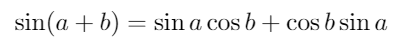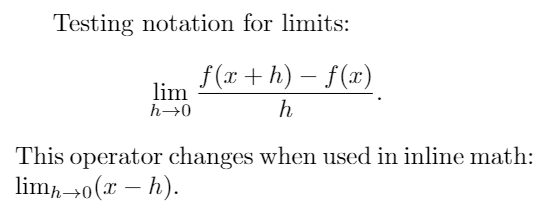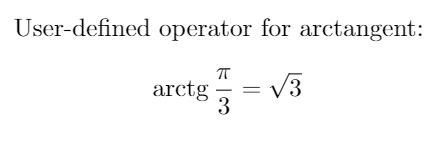Showing first {{hits.length}} results of {{hits_total}} for {{searchQueryText}}{{hits.length}} results for {{searchQueryText}}

검색 결과 없음

Characters in mathematical mode are usually shown in italics, but sometimes especial function names require different formatting, this is accomplished by using operators defined in LaTeX. Contents 1 Introduction 2 Operators in different contexts 3 Defining your own operators 4 Reference guide 5 Further reading Introduction Trigonometrical functions, logarithms, and some others can be written in a document by means of some special commands. Examples of mathematical operators $\sin(a + b ) = \sin(a)\cos(b) + \cos(a)\sin(b)$The commands will print the name of the function in Roman characters instead of italics.   Open an example in Overleaf Operators in different contexts Some operators can take parameters that are handled in a special way, for instance, limits. Testing notation for limits $\lim_{h \rightarrow 0 } \frac{f(x+h)-f(x)}{h}$ This operator changes when used alongside text $$\lim_{x \rightarrow h} (x-h)$$.Notice how the limit declaration can include a subscript. See the reference guide for a complete list of available operators. Some languages may add or change some commands, check the main page for language-specific articles.   Open an example in Overleaf Defining your own operators If you need to add a personalized operator to be displayed in Roman font instead of italics use \DeclareMathOperator \documentclass{article} \usepackage{amssymb} \usepackage{amsmath} \DeclareMathOperator{\Mr}{M_{\mathbb{R}}} \begin{document} User-defined operator for matrices with Real entries $x \in \Mr$ \end{document}The command \DeclareMathOperator takes two parameters, the first one is the name of the new operator and the second one is the text to be displayed. For this command to work you have to import the package amsmath in the preamble with \usepackage{amsmath} The command can be slightly modified if you need that your defined operator uses subscripts, as the \lim operator, in such case use \DeclareMathOperator*.   Open an example in Overleaf Reference guide Complete list of mathematical operators Operator Renders as \cos $\cos$\csc $\csc$\exp $\exp$\ker $\ker$\limsup $\limsup$\min $\min$\sinh $\sinh$\arcsin $\arcsin$\cosh $\cosh$\deg $\deg$\gcd $\gcd$\lg $\lg$\ln $\ln$\Pr $\Pr$\sup sup \arctan $\arctan$\cot cot \det $\det$\hom $\hom$\lim lim \log $\log$\sec sec \tan $\tan$\arg $\arg$\coth $\coth$\dim $\dim$\liminf $\liminf$\max $\max$\sin $\sin$\tanh $\tanh$Further reading For more information see Mathematical expressions Subscripts and superscripts Fractions and Binomials Spacing in math mode Integrals, sums and limits Display style in math mode The not so short introduction to LaTeX2ε